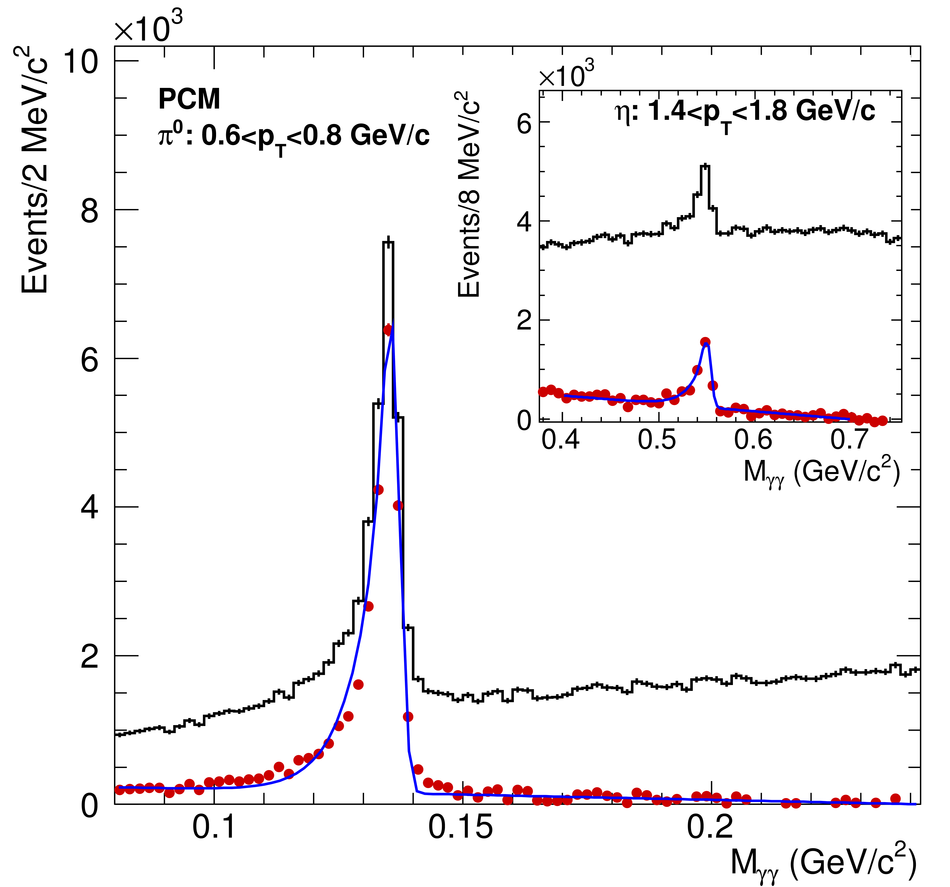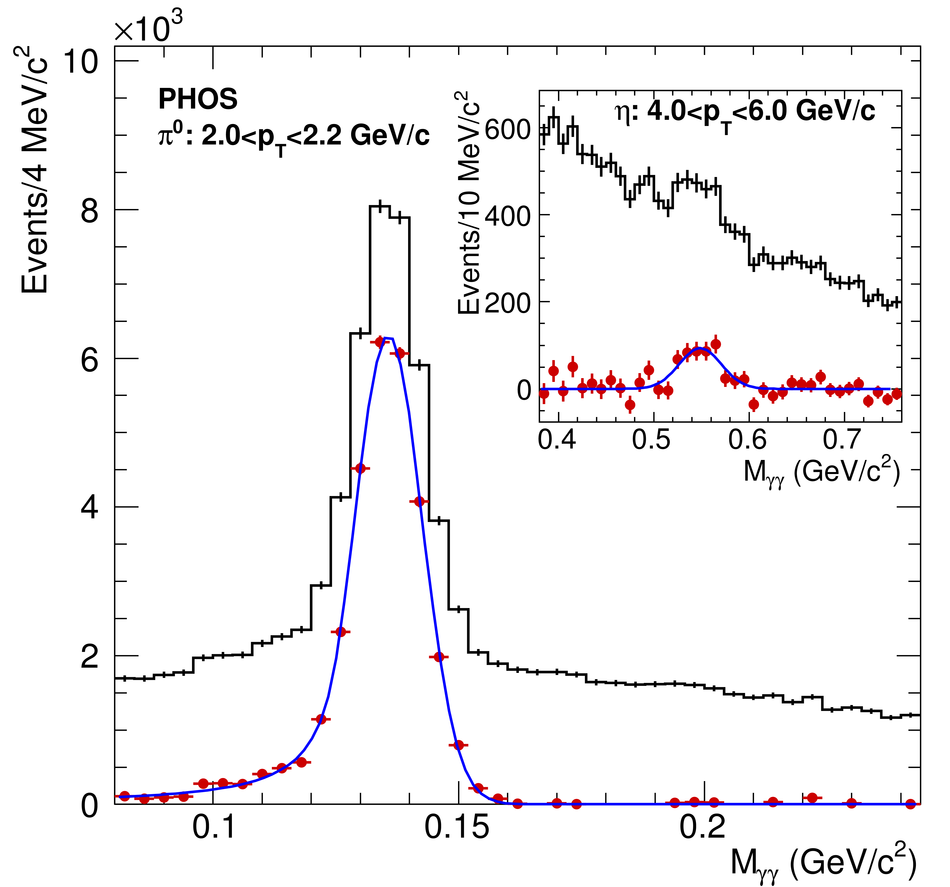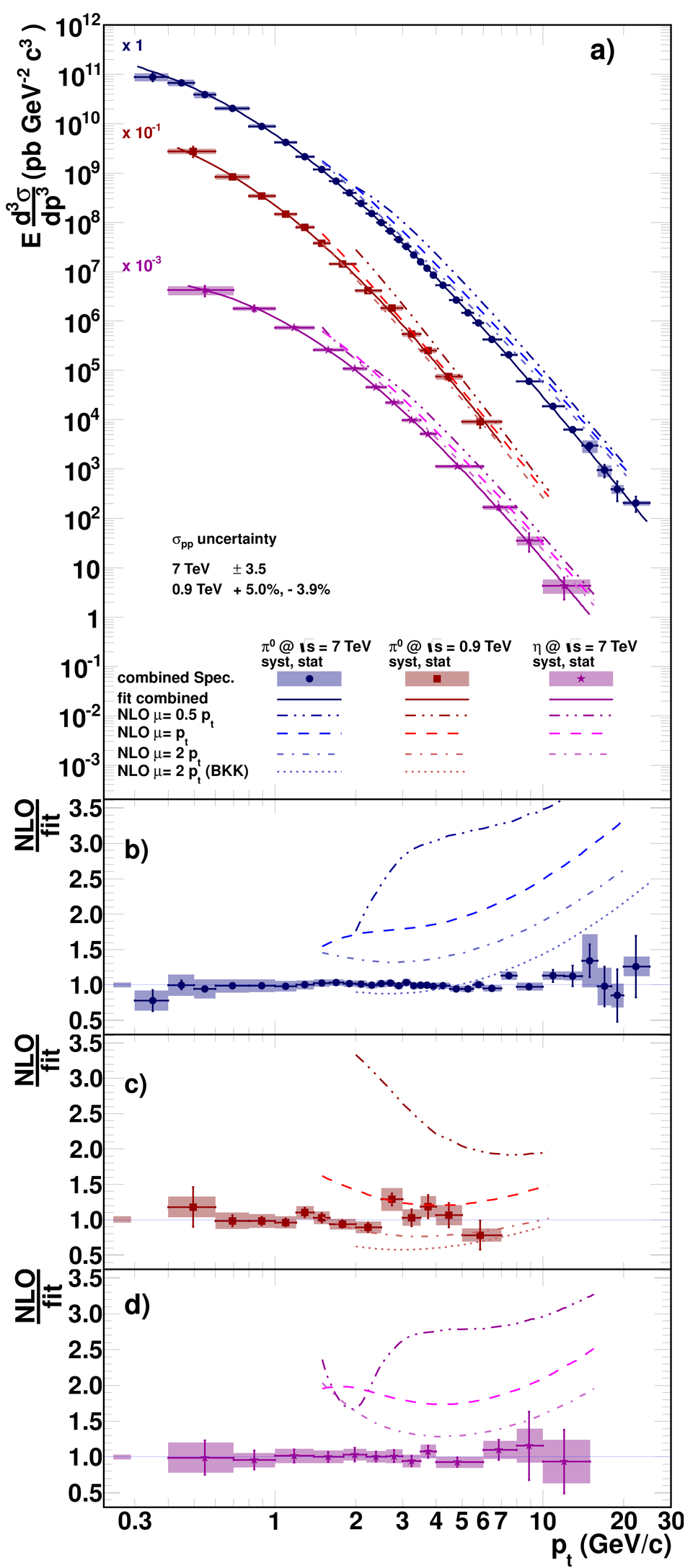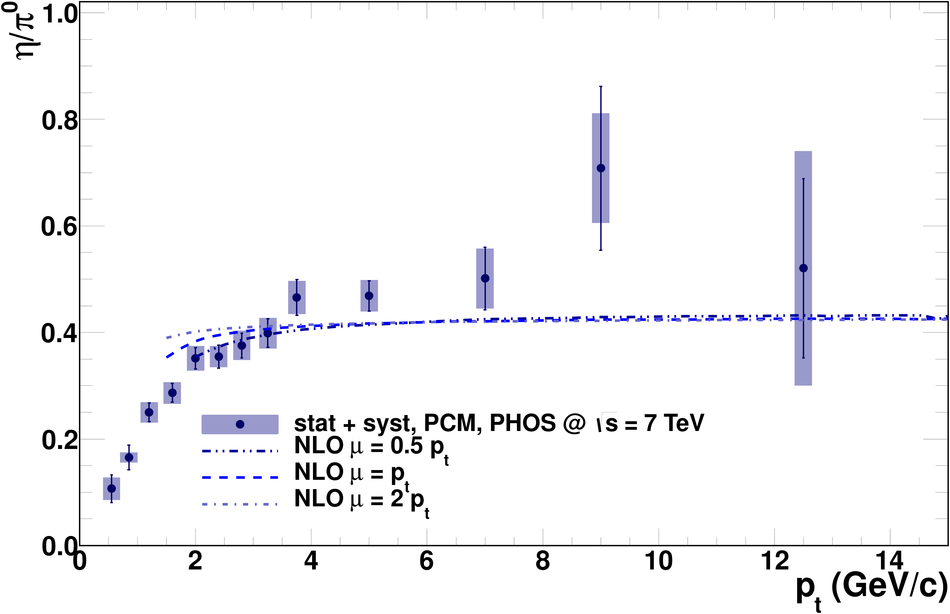# Neutral pion and $η$ meson production in proton-proton collisions at $\sqrt{s}=0.9$ TeV and $\sqrt{s}=7$ TeV

The first measurements of the invariant differential cross sections of inclusive $\pi^0$ and $\eta$ meson production at mid-rapidity in proton-proton collisions at $\sqrt{s}=0.9$ TeV and $\sqrt{s}=7$ TeV are reported. The $\pi^0$ measurement covers the ranges $0.4<~p_T<~7$ GeV/$c$ and $0.3<~p_T<~25$ GeV/$c$ for these two energies, respectively. The production of $\eta$ mesons was measured at $\sqrt{s}=7$ TeV in the range $0.4<~p_T<~15$ GeV/$c$. Next-to-Leading Order perturbative QCD calculations, which are consistent with the $\pi^0$ spectrum at $\sqrt{s}=0.9$ TeV, overestimate those of $\pi^0$ and $\eta$ mesons at $\sqrt{s}=7$ TeV, but agree with the measured $\eta/\pi^0$ ratio at $\sqrt{s}=7$ TeV.

Figures

## Figure 1

 Invariant mass spectra in selected $pt$ slices in PCM (left) and PHOS (right) in the $\pi^0$ and $\eta$ meson mass regions. The histogram and the bullets show the data before and after background subtraction, respectively. The curve is a fit to the invariant mass spectrum after background subtraction.## Figure 2

 Reconstructed $\pi^0$ peak width (a) and position (b) as a function of $\pt$ in pp collisions at $\sqrt{s}=7$ TeV in PHOS and in the photon conversion method (PCM) compared to Monte Carlo simulations. The horizontal line in (b) indicates the nominal $\pi^0$ mass.## Figure 3

 a) Differential invariant cross section of $\pi^0$ production in pp collisions at $\sqrt{s}=7$ TeV (circles) and 0.9 TeV (squares) and of $\eta$ meson production at $\sqrt{s}=7$ TeV (stars). The lines and the boxes represent the statistical and systematic error of the combined measurement respectively. The uncertainty on the pp cross section is not included NLO pQCD calculations using the CTEQ6M5 PDF and the DSS (AESS for $\eta$ mesons) FF for three scales $\mu=0.5\pT$, $1\pT$ and $2\pT$ are shown. Dotted lines in panels b) and c) correspond to the ratios using the BKK FF. Ratio of the NLO calculations to the data parametrisations are shown in panels b), c) and d). The full boxes represent the uncertainty on the pp cross sections.## Figure 4

 Ratio of the two independent $\pi^0$ meson measurements to the fit of the combined normalized invariant production cross section of $\pi^0$ mesons in pp collisions at $\sqrt{s}=7$ TeV.## Figure 5

 $\eta/\pi^0$ ratio measured in pp collisions at $\sqrt{s}=7$ TeV compared to NLO pQCD predictions.